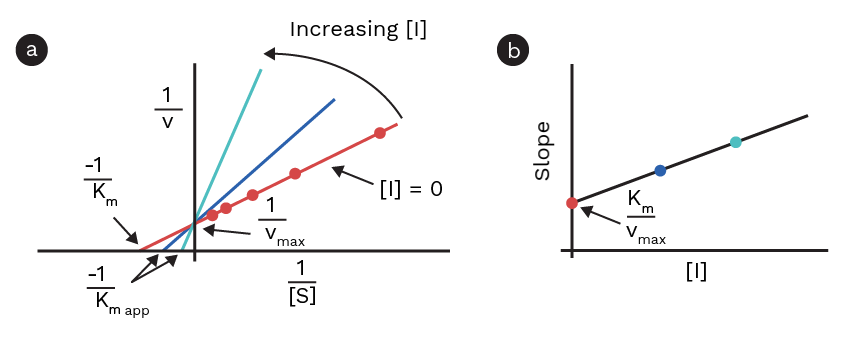The double-reciprocal equation for competitive inhibition is as follows:

1/V0 = 1/Vmax + Km • α/Vmax • 1/[S] where α = 1 + I/ KI

Based on this equation, a double-reciprocal plot should give a straight line, with the intercept 1/ Vmax and slope Km • α / Vmax. Different Lineweaver-Burk plots with varying inhibitor concentrations should, therefore, give different slopes (because α increases with the inhibitor concentration), but the same y-intercept. This means, that Vmax at different concentrations of a competitive inhibitor is unchanged; however, the apparent Km, Km(app) (Km(app) = Km • α), differs. If double-reciprocal plots of 1/V0 against 1/[S] with varied inhibitor concentrations yield straight lines, with different slopes, but with the same y-intercept, the inhibitor is competitive (Figure 1) .Figure 1: Figure a; Lineweaver-Burk plot showing competitive inhibition. Figure b; Shows slopes of each linear regression plotted against the inhibitor concentration.

## Calculating KI, Km, and Vmax

If the inhibitor is competitive, only 1 inhibitor constant needs to be calculated. To calculate the inhibitor constant, several assays with different inhibitor concentrations must be conducted. Each of the resulting datasets should be plotted and the slopes and y intercepts can be determined by linear regression. From these fits, Vmax can be calculated as the reciprocal of the y-intercept. If none of the kinetic parameters have been determined, this linear fit does not provide enough information to determine KI and Km. To determine these parameters, it is necessary to plot the ‘’slopes’’ from the different assays against the inhibitor concentration. Thus based on the following equation:

Slope competetive = Km • α/Vmax = Km/Vmax + Km/Vmax • 1/KI • [I]

This plot should therefore also result in a straight line with the intercept Km / Vmax, and slope (Km / Vmax ) • 1 / KI. Thus, KI can be calculated by dividing the y-intercept with the slope. Because Vmax has already been calculated, Km can be calculated from the y-intercept of this fit, by multiplying this intercept with Vmax.

## Steps of calculating the kinetic parameters when using a competitive inhibitor

Prepare Lineweaver-Burk plots of the kinetic data and fit the data using linear regression (1 fit per inhibitor concentration). The y-intercepts of the Lineweaver-Burk plots at different inhibitor concentrations should be the same (or at least close). Take the reciprocal to the y-intercept; this is Vmax. Plot the slopes of each of these lines as a function of the inhibitor concentration in a new plot, and fit this plot using linear regression. To calculate Km, multiply the y-intercept of this line with Vmax. To calculate KI, divide the y-intercept of this line with the slope.

1. Lehninger, Albert L.; Nelson, David L.; Cox, Michael M. (2008). Principles of Biochemistry (5th ed.). New York, NY: W.H. Freeman and Company. ISBN 978-0-7167-7108-1.

Theory overview